GMAT Question of the Day - Daily to your Mailbox; hard ones only

 It is currently 11 Dec 2019, 17:54### GMAT Club Daily Prep

#### Thank you for using the timer - this advanced tool can estimate your performance and suggest more practice questions. We have subscribed you to Daily Prep Questions via email.

Customized
for You

we will pick new questions that match your level based on your Timer History

Track

every week, we’ll send you an estimated GMAT score based on your performance

Practice
Pays

we will pick new questions that match your level based on your Timer History

#### Not interested in getting valuable practice questions and articles delivered to your email? No problem, unsubscribe here.# GMAT Quant DS - 3 Mistakes Students Make in Number Properties Question

Author Message
TAGS:

### Hide Tags

e-GMAT RepresentativeV
Joined: 04 Jan 2015
Posts: 3158
GMAT Quant DS - 3 Mistakes Students Make in Number Properties Question  [#permalink]

### Show Tags

1
13
Added the PDF of the article at the end of the post!GMAT Quant DS - 3 Mistakes Students Make in Number Properties Questions - Part I

After reading this article you will recognize three common reasons why you make a mistake and the steps you can take to read or eliminate the same.

Agenda of the Article
A major part of solving a DS question correctly involves inferring the information from the question stem…
• And, this is an area where students typically falter.

To help you, we will discuss three major mistakes that students make while applying inferences in a DS question.
These 3 mistakes are:
1. Drawing incorrect inference from the question stem.
2. Drawing incomplete inference from the question stem.
3. Faltering while applying the inferences.

We will take one question each to discuss the above 3 types of mistakes.
• Note: Since these questions are e-GMAT practice quiz questions, we will also provide the accuracy and total number of forum queries for each question.

Let us discuss the first mistake.

Mistake 1: Drawing Incorrect Inference from the Question Stem

Many times, a student knows exactly how to solve a question correctly – he knows all the concepts, he even knows how to go about solving the question, yes makes a mistake by drawing incorrect inference(s) from the question stem. The most common reason for the same - rushing through while reading the question stem.
In mistake 1, we will look at one e-GMAT Practice question where students have made this type of mistake.
Let us look at the question first.

Question 1

x, y, and z are positive integers, where x is an odd number and z = $$x^2$$ + $$y^2$$ + 4. Is $$y^2$$ divisible by 4?
1. z = 8k - 3
2. (z – x + 1) = 2q + 1

(For e-GMAT students: This question is present in the Even-Odd practice quiz of Number properties module)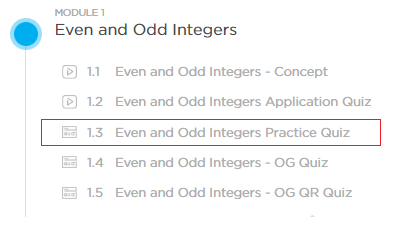Accuracy of the question: 55.10%

Here is the number of responses against each option and the corresponding percentage: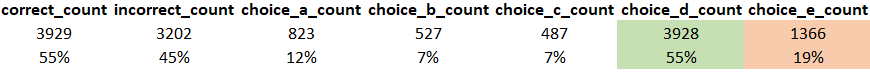Total relevant forum post queries posted for this question: 14
• We know that 45% of the students got this question wrong and out of this 45%, every 2 out of the 5 students knew all the concepts and still made the mistake.

So, let us understand this question first!

Given:
• x, y, and z are positive integer and x is an odd number.
• z = $$x^2$$ +$$y^2$$ + 4

Inference from the given information:

• z = Odd + $$y^2$$ + Even
• z = Odd + $$y^2$$ (As Odd + Even = Odd)

To Find:

We need to find whether $$y^2$$ is divisible by 4 or not.

Inference from what we need to find:

Now, let us first look at one incorrect inference.

Student’s Mistake – Incorrect Inference
Notice the highlighted part of this query.Now, is there anything wrong in this inference drawn by the student?
• It is actually partially incorrect.
o The reason is that student is considering only those values of y that are divisible by 4.
o What if y is divisible by 2 only and not divisible by 4?
 For example, what if y = 2 or 6 or 10?
• Is $$y^2$$ = 4 or 36 or 100 not divisible by 4?
• It is.

Correct Inference

Hence, the correct inference is that if y is divisible by 2 then $$y^2$$ will be divisible by 4.
• Or, essentially if y is even then $$y^2$$ is divisible by 4.

So, if you focus on processing information in the correct manner then you can get the question correct.
• The best thing is you don’t need to learn any new concepts, it is only about developing good habits i.e. following step by step and methodical process.

How to Avoid Drawing Incorrect Inferences

1. The first reason why students make this mistake is because drawing inferences from question stem is not a part of their process to solve a DS question.
a. Basically, they start to draw inferences from the statements and that is how they make this mistake.
b. So, make sure to include this step in you process.

2. Secondly, drawing inferences from the question stem may be part of a student’s process of solving a question, yet the student tends to rush while doing the same. Therefore:
a. The student draws incomplete inferences.
b. Or the student is unable to draw any inference at all.

3. Now there are scenarios in which the student knows the concepts and also spends enough time to draw inferences, and yet is unable to draw the correct inference because the student does not have the depth/capability to draw inferences.
a. If such is the case, then such questions present excellent learning opportunities. Bookmark this question and revise it at least twice. Doing so will improve your ability to infer and you will make fewer similar mistakes.

Mistake 2: Drawing Incomplete Inference from the Question Stem
This is the second type of mistake where students infer incomplete information from the question stem.
To understand the same, let us look at another e-GMAT question.

Question 2

The number x is a positive odd integer. If the unit digit of $$x^3$$ is subtracted from the unit digit of $$x^2$$, it results in 0. What is the unit digit of the number x + 7?
1. The unit digit of the product of 105 and x is 5.
2. When x is divided by 5, it leaves no remainder.

(For e-GMAT students: This question is present in units digit practice quiz of Number properties module)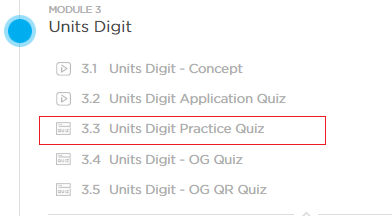Accuracy of the question: 44.47%

Here is the number of responses against each option and the corresponding percentage: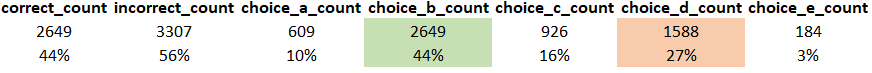Total relevant forum post queries posted for this question: 14
• Out of the 55% who got this question wrong, every 1 out of 3 students knew the concepts and still got the question wrong.

Let us understand why this happened.

Given:

• x is a positive odd integer.
• When the unit digit of $$x^3$$ is subtracted from the unit digit of $$x^2$$, it results in 0.

Inference from the given information:

Let us look at one incomplete inference first.

Student’s Mistake: Incomplete Inference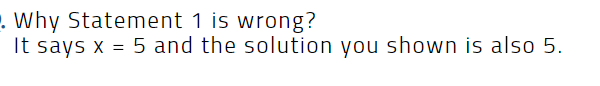This student thinks that 105 is ending with 5 and its multiplication with another number is also ending with 5.
• So, he does some calculation in the back of his mind that:
o 5*5 ends with 5
o 25* 5 also ends with 5
o 125* 5 also ends with 5.
• Therefore, x should be 5.

Note, the student here did spend the time to draw this inference. He also applied the right concepts. Yet, despite this, the inference he drew was incomplete.
Therefore, why don’t you spend some time and try to draw the correct inference.

Correct Inference
Since the subtraction of units digits of $$x^3$$ and $$x^2$$ are resulting in 0, we can say that their units digits are identical (or equal).
• Units digit of $$x^3$$ = Units digit of $$x^2$$
Hence, we can say that the cyclicity of units digit of x is 1.
Therefore, the possible units digit of odd integers with cyclicity 1 can be either 1 or 5 and not just 5.

For example:
• For x = 11, last digit of (105 × 11) = last digit of (5 × 1) = 5.
• For x = 31, last digit of (105 × 31) = last digit of (5 × 1) = 5.
• For x = 35, last digit of (105 × 35) = last digit of (5 × 5) = 5.
• For x = 65, last digit of (105 × 65) = last digit of (5 × 5) = 5.

So, the student made two mistakes.
1. He forgot to consider that the units digit of x can also be 1 – which is an incomplete inference.
2. He also relied on number substitution which gives wrong answers in many questions.
a. The reason is simple – you take only one value and do not think for other possible values.
b. As we saw in this question, last digit 5 works but it is not the only value of the last digit of x that works.

And, do you know that test makers also form such questions by keeping in minds these traps?
Want to see how…?

Let us read statement 1 again.

Statement 1: The unit digit of the product of 105 and x is 5.
• Units digit of (105 × x) = 5

For units digit of x =1 or 5, the units digit of (105 × x) is 5. For example:
1. Units digit of (105 × 11) = Units digit of (5 × 1) = 5.
2. Units digit of (105 × 25) = Units digit of (5 × 5) = 5.

If the student draws an incomplete inference and considers only 5 or only 1, then he will think that statement 1 is sufficient to answer and that is how he will fall into the trap.
• And, the only way to ensure that one does not fall into these kinds of traps is to completely and correctly infer all the information present in the question.

How to avoid drawing incomplete inferences
• You can avoid making incomplete inferences by following a proper step by step and methodical approach in every DS question.
• As in this question, we first found that cyclicity of units digit of x is 1 and then we found two possible values of x rather than directly coming to a value without proper analysis.
• This ensures that we are not missing out any inference that might be useful to solve a question correctly.

So, we have learned to avoid the 2 most common mistakes in DS questions.
We will discuss “Mistake 3: Faltering while applying the inferences” in the next article.

1. We discussed how important it is to draw inferences from question stem.
a. Please understand that the question stem provides important information that needs to be processed and inferred first.
b. If this step is still not in your process, do add this and your accuracy will improve.

2. Don’t rush through reading the question stem because you might draw incomplete and/or incorrect inferences.
a. Make a conscious effort to not rush through things. To start off, you can even assign yourself 45 sec – 1 min to process information and with practice, this process will happen automatically every time you will solve a question. Similarly, the time required to infer information will reduce with practice as well.

3. There are some questions in which you spend the time to draw inference from the question stem and yet are make a mistake. Bookmark these questions; treat them as a learning opportunity.
a. Make notes and such questions a couple of times and your propensity to make such mistakes will reduce.

Want to read more articles like this?

If you liked this article, then I am sure that you want to read some more articles like this.

To read all our article go here:Must Read Articles and Practice Questions to score Q51

You will also get 50+ practice questions, across various topics, to learn from.

Happy learning.

Attachments3Mistakes_Number Properties – Part I.pdf [476.24 KiB]

_________________

Originally posted by EgmatQuantExpert on 15 Mar 2019, 13:32.
Last edited by EgmatQuantExpert on 24 Mar 2019, 23:19, edited 8 times in total.
InternB
Joined: 29 Aug 2016
Posts: 24
Re: GMAT Quant DS - 3 Mistakes Students Make in Number Properties Question  [#permalink]

### Show Tags

thanks
e-GMAT RepresentativeV
Joined: 04 Jan 2015
Posts: 3158
Re: GMAT Quant DS - 3 Mistakes Students Make in Number Properties Question  [#permalink]

### Show Tags

e-GMAT RepresentativeV
Joined: 04 Jan 2015
Posts: 3158
Re: GMAT Quant DS - 3 Mistakes Students Make in Number Properties Question  [#permalink]

### Show Tags

Reference Question 1

If x, y, and z are positive integers, where x is an odd number and $$z = x^2 + y^2 + 4$$. Is $$y^2$$ divisible by 4?
I. z = 8k - 3 where k is a positive integer
II. (z - x + 1) = 2q + 1, where q is a positive integer

Solution

Steps 1 & 2: Understand Question and Draw Inferences
We are given that
• x, y and z are positive integers.
• Also, x is odd
• And, $$z= x^2 + y^2 + 4$$

By rearranging the terms, we get:
$$y^2 = z – x^2 – 4$$ …………. (1)

The question asks us whether $$y^2$$ is divisible by 4 or not. If y is divisible by 2, then $$y^2$$ will be divisible by 4; else it will not be.
And if y is divisible by 2, this means that y is even.
• Thus, the question is indirectly asking us: is y even?
y will be even if the right-hand side of Equation 1 is even. So, let’s analyze the condition in which this will be so.

Now, we are given that x is an odd number. This means that $$x^2$$ is also odd (Odd * Odd = Odd)
So, in the part $$(x^2 – 4)$$, we are subtracting an even number from an odd number. Think of what happens when we subtract 4 from 9. We get 5, which is an odd number. So, here too, the result of subtracting 4 from $$x^2$$ will be an Odd number (Please note that the result may be positive or negative. We are not concerned with that. All that we want to know is whether the result will be even or odd)

So, from Equation 1 we get:
• $$y^2$$ = z – Odd number . . . (2)

Now,
• Even +/- Odd = Odd (Think: 4 + 1 = 5)
• And, Odd +/- Odd = Even (Think: 3 + 1 = 4)

Thus, from equation (2),
It is clear that $$y^2$$ will be even only if z is an odd number
• y will be even only if z is an odd number
Our task is to determine if z is odd or not.

Step 3: Analyze Statement 1
Statement (1) says: z = 8 * k – 3

Now, irrespective of whether k is even or odd,
• 8 * k = even number
• 8k – 3 = Even number - odd number = Odd Number (Think: 8 – 3 = 5)

So, the analysis of statement (1) tells us that z is an odd number.
Since we have been able to determine that z is an odd number, this means that y is an even number.
Thus, Statement (1) alone is sufficient to answer the question: is y even?

Step 4: Analyze Statement 2
Statement (2) says: (z - x + 1) = 2q + 1, where q is a positive integer
• 2q+1 = odd
Hence, z – x + 1 = odd
z – x = Odd – 1 = Odd – Odd = Even
So, z = x + Even number
z = Odd + Even = Odd
Thus, z is an odd number

Hence, y is an even number
Thus, Statement 2 alone is sufficient to answer the question: is y even?

Step 5: Analyze Both Statements Together (if needed)
Since we got unique answers in step 3 and 4, so this step is not required

_________________
e-GMAT RepresentativeV
Joined: 04 Jan 2015
Posts: 3158
Re: GMAT Quant DS - 3 Mistakes Students Make in Number Properties Question  [#permalink]

### Show Tags

Reference Question 2

The number x is a positive odd integer. If the unit digit of $$x^3$$ is subtracted from the unit digit of $$x^2$$, it results in 0. What is the unit digit of the number x + 7?
I. The unit digit of the product of 105 and x is 5.
II. When x is divided by 5, it leaves no remainder.

Solution

Steps 1 & 2: Understand Question and Draw Inferences
We are given that
• x is a positive odd number.
• Also, (The unit digit of $$x^2$$) – (the unit digit of $$x^3$$) = 0
o This implies, the unit digits of $$x^2$$ and $$x^3$$ are same.

So, we know two things about the number x:
• It’s an odd number.
• The cyclicity of the unit digit of x is 1 since the unit digits of $$x^2$$ and $$x^3$$ are same.

Now, in odd numbers, we know that the cyclicity of only 1 and 5 is 1. So, the unit digit of x is either 1 or 5.
• So, we have to find whether the unit digit of x is 1 or 5.
o Because once we get to know the unit digit of x, we can add 7 to it and find the unit digit of x + 7.

Step 3: Analyze Statement 1
Statement 1 says: The unit digit of the product of 105 and x is 5.

Now, we know that the unit digit of the product of 105 and x will be the product of the unit digit of 105 and the unit digit of x.
The unit digit of 105 is 5, and the unit digit of x is either 1 or 5. So, let’s consider both the cases:

Case 1: The unit digit of x is 1.
• So, the unit digit of 105x = 5*1 = 5
Thus, if the unit digit of x is 1, the unit digit of 105x will be 5.

Case 2: The unit digit of x is 5.
• So, the unit digit of 105x = 5 * 5 = The unit digit of 25 = 5
Thus, if the unit digit of x is 5, the unit digit of 105x will be 5.

So, we can’t determine the unit digit of x from this statement.
Hence, statement (1) is not sufficient to answer the question: What is the unit digit of x + 7?

Step 4: Analyze Statement 2
Statement 2 says: When x is divided by 5, it leaves no remainder.

Now, we know that the multiple of 5 can only have 0 or 5 at the units place. Also, x is an odd number.
• So, $$\frac{x}{5}$$ will not leave any remainder only if the unit digit of x is 5.
So, we know that the unit digit of x is 5.

Now, since we know the unit digit of x we can find the unit digit of x + 7.
So, statement (2) alone is sufficient to answer the question: What is the unit digit of x + 7?

Step 5: Analyze Both Statements Together (if needed)
Since statement (2) alone is sufficient to answer the question, we don’t need to perform this step.

_________________
InternB
Joined: 29 Aug 2016
Posts: 24
Re: GMAT Quant DS - 3 Mistakes Students Make in Number Properties Question  [#permalink]

### Show Tags

Hi, Can you share part 2 articles?
e-GMAT RepresentativeV
Joined: 04 Jan 2015
Posts: 3158
Re: GMAT Quant DS - 3 Mistakes Students Make in Number Properties Question  [#permalink]

### Show Tags

Hey calculusbro,

We have not posted the second article yet.
We will surely notify you once we post the next article.

Till then, you read more of our articles by checking this link: https://gmatclub.com/forum/e-gmat-quant ... l#p1525390

Regards,
Ashutosh
_________________
e-GMAT RepresentativeV
Joined: 04 Jan 2015
Posts: 3158
Re: GMAT Quant DS - 3 Mistakes Students Make in Number Properties Question  [#permalink]

### Show Tags

Hey calculusbro,

We have not posted the second article yet.
We will surely notify you once we post the next article.

Till then, you can read more of our articles by checking this link: https://gmatclub.com/forum/e-gmat-quant ... l#p1525390

Regards,
Ashutosh
_________________Re: GMAT Quant DS - 3 Mistakes Students Make in Number Properties Question   [#permalink] 31 Jul 2019, 05:07
Display posts from previous: Sort by

# GMAT Quant DS - 3 Mistakes Students Make in Number Properties Question